## KMP 算法详细解析

Published on 2015-11-14

## 字符串匹配算法

KMP算法由D.E.Knuth，J.H.Morris和V.R.Pratt同时发现，因此人们称它为克努特——莫里斯——普拉特操作（简称KMP算法）

## 朴素的匹配方法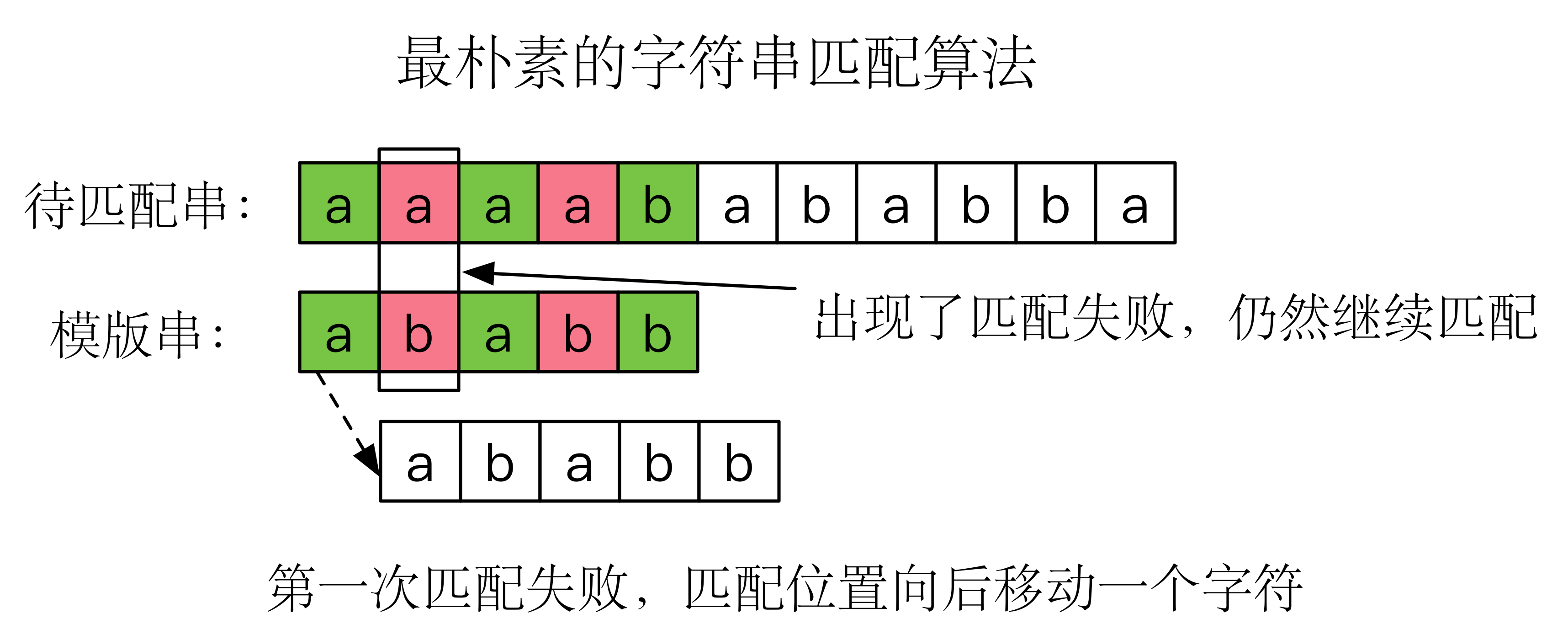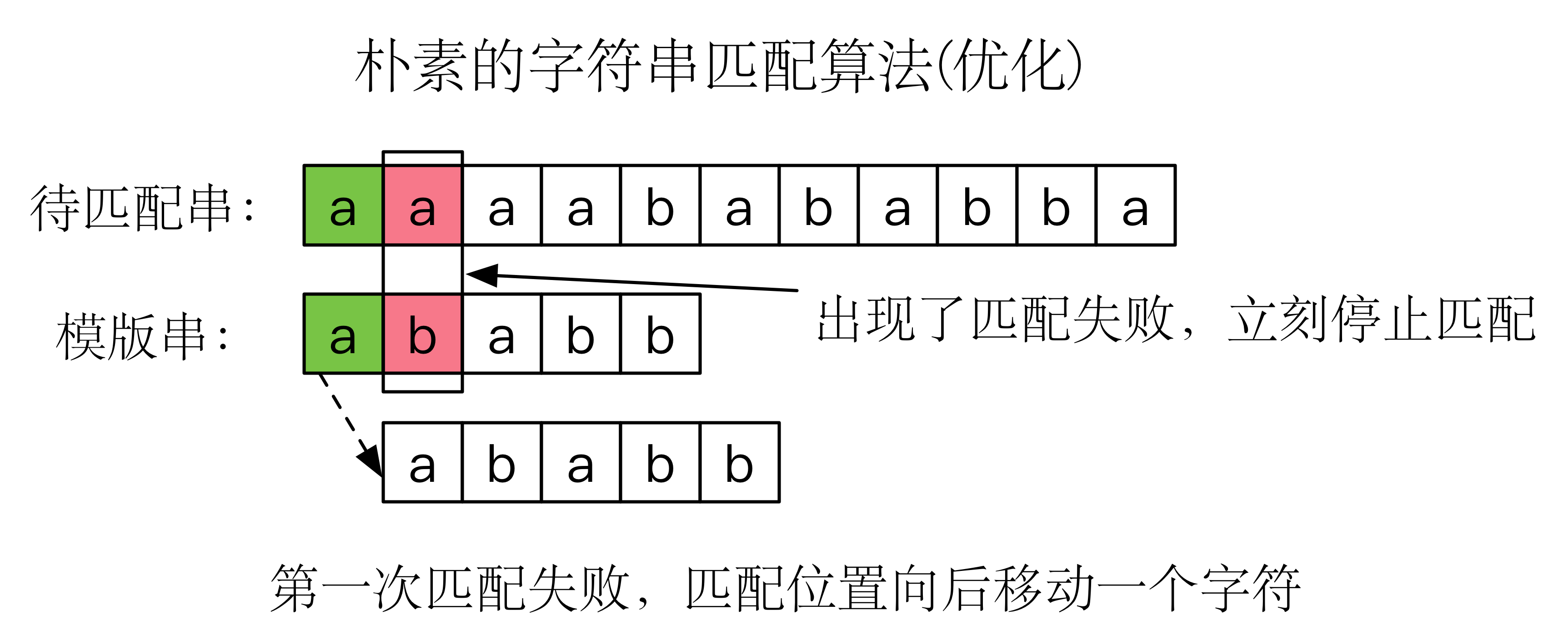## KMP算法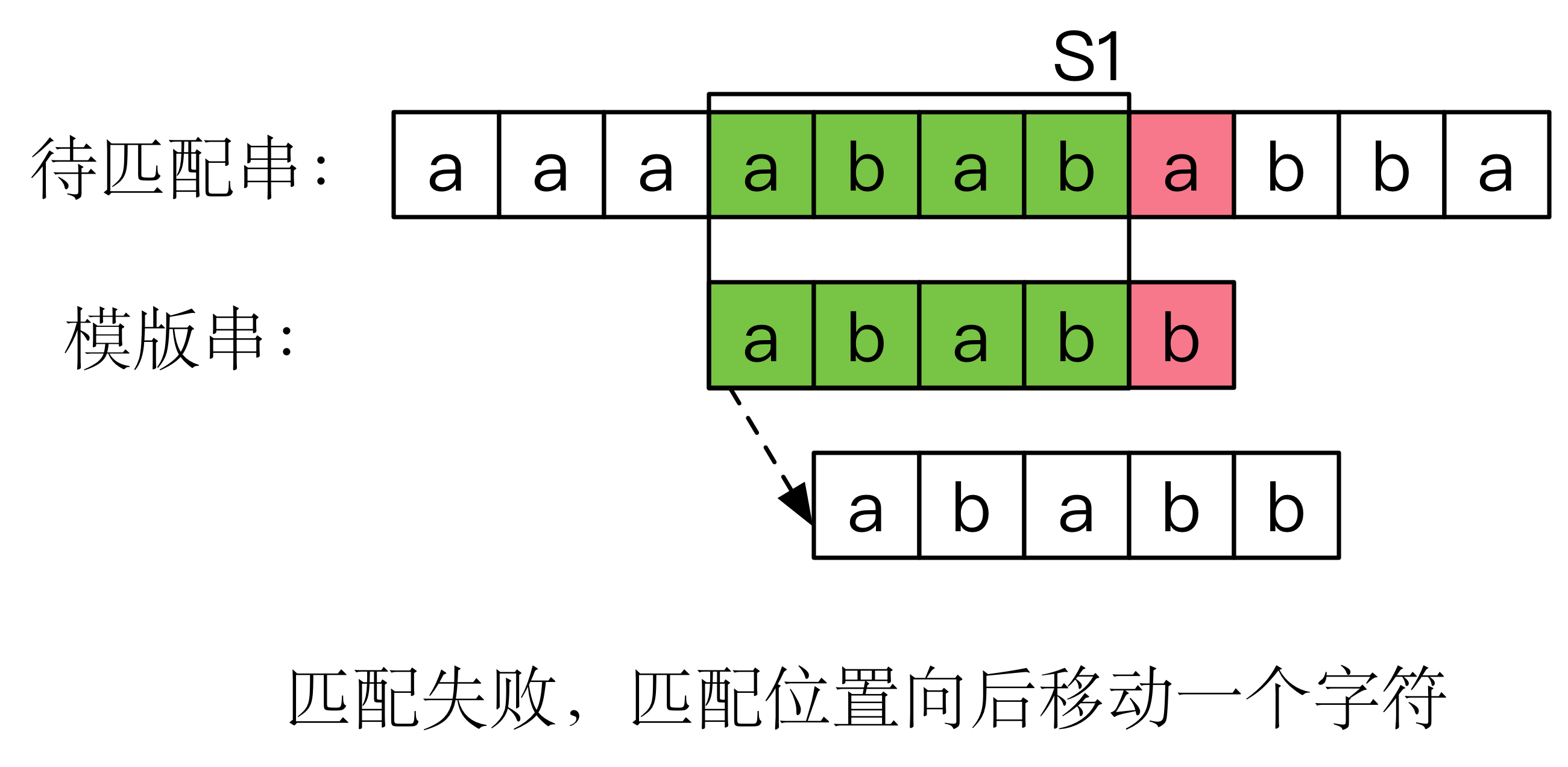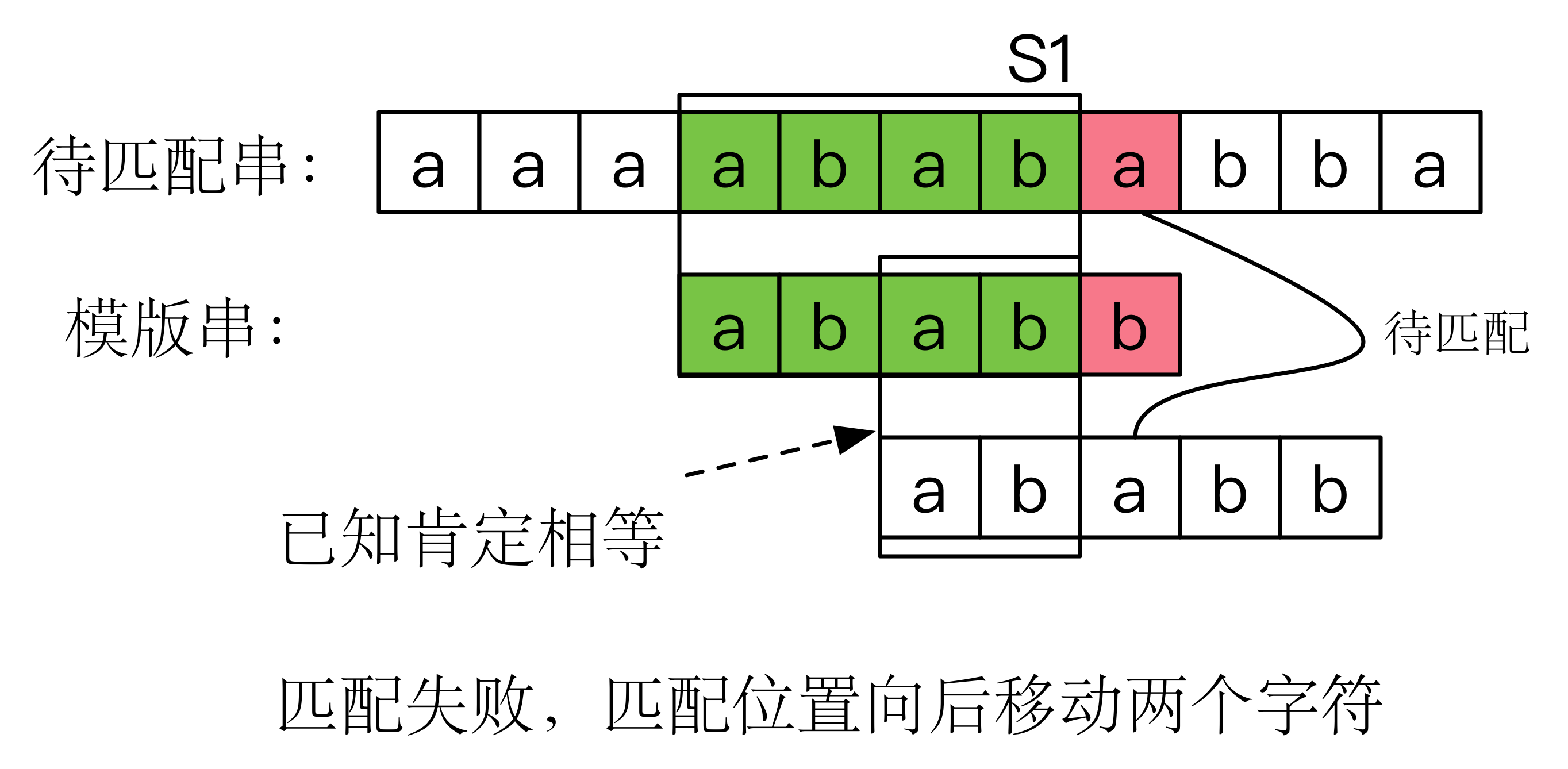$j - f(j)$

### 算法实现

void findstr(const char *str, const char *temp, int *f) {
int n = strlen(str), m = strlen(temp);
process(temp, f); //预处理得到失配表
int j = 0; //j表示当前模版串的待匹配位置
for(int i = 0; i < n; ++i) {
while(j && str[i] != temp[j]) j = f[j]; //不停的转移，直到可以匹配或者走到0
if(str[i] == temp[j]) j++; //如果相等，模版串中待匹配位置可以移一位了。
if(j == m) printf("%d\n", i - m + 1);
}
}



KMP 算法的精髓就蕴藏在下图中：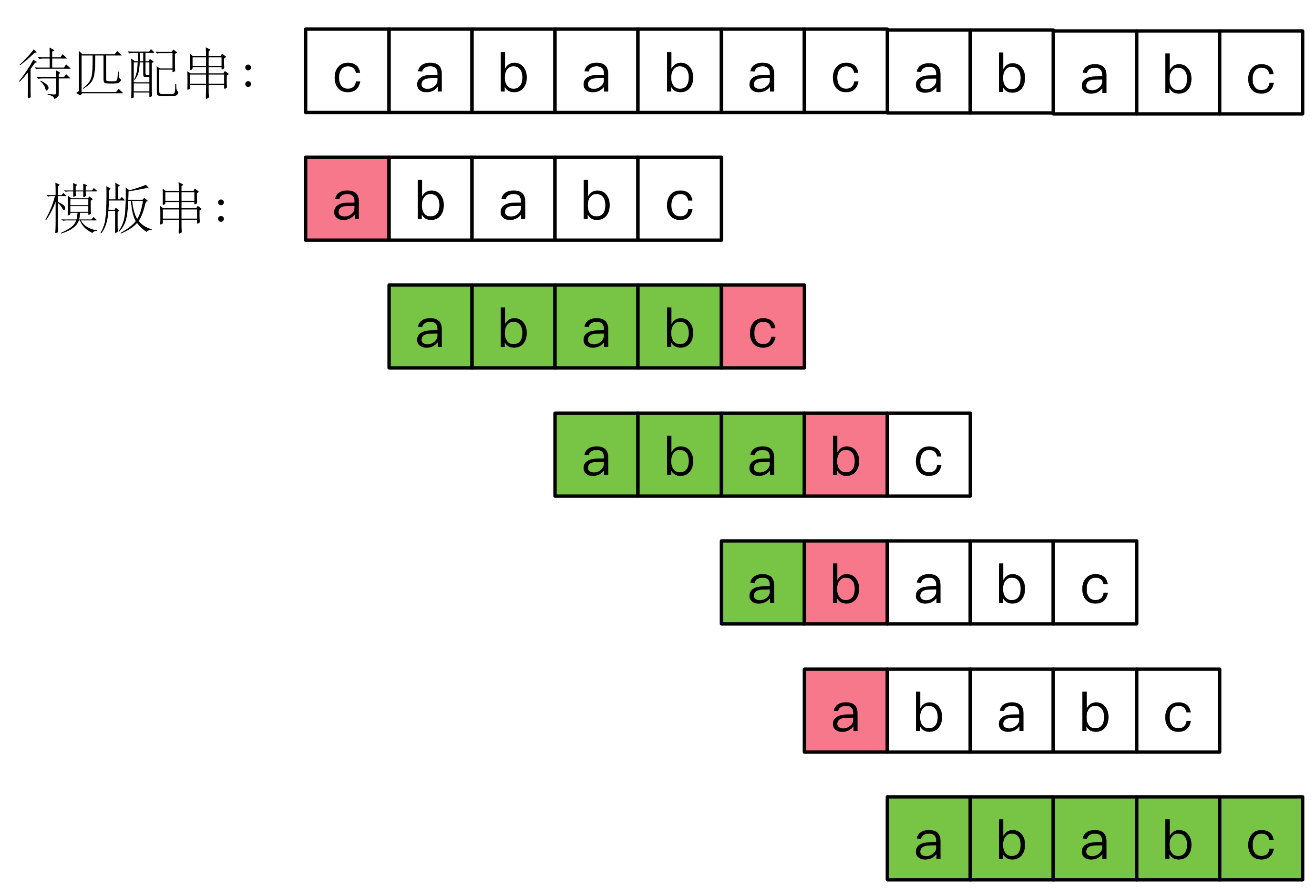### 计算失配表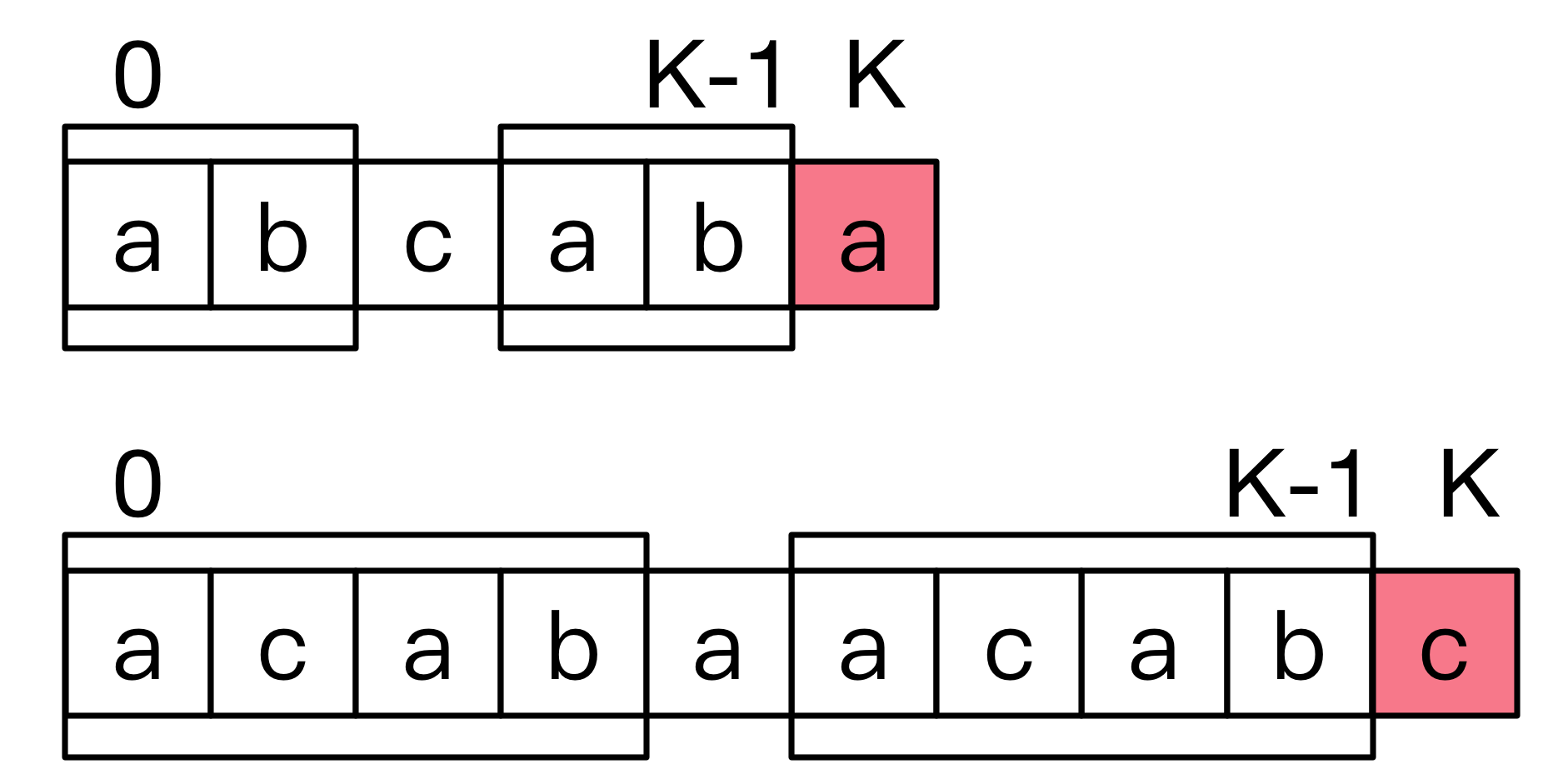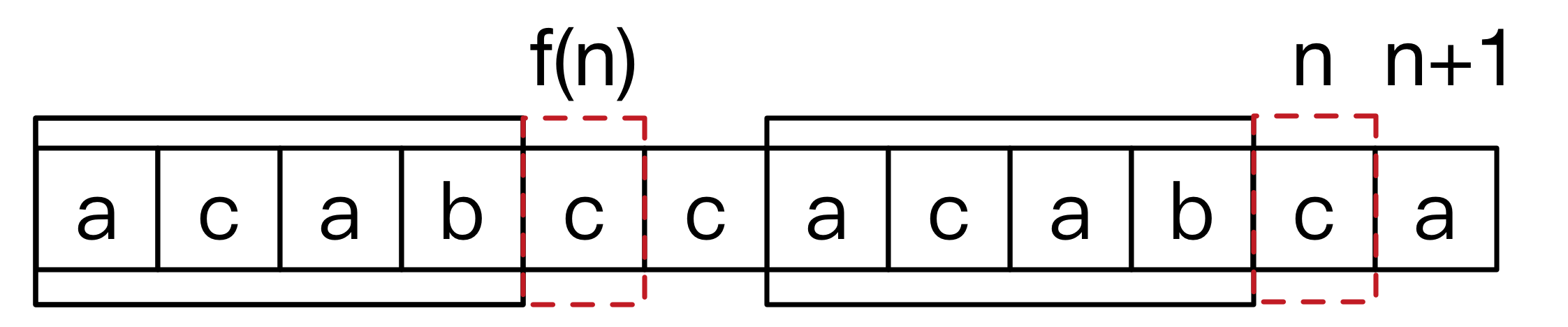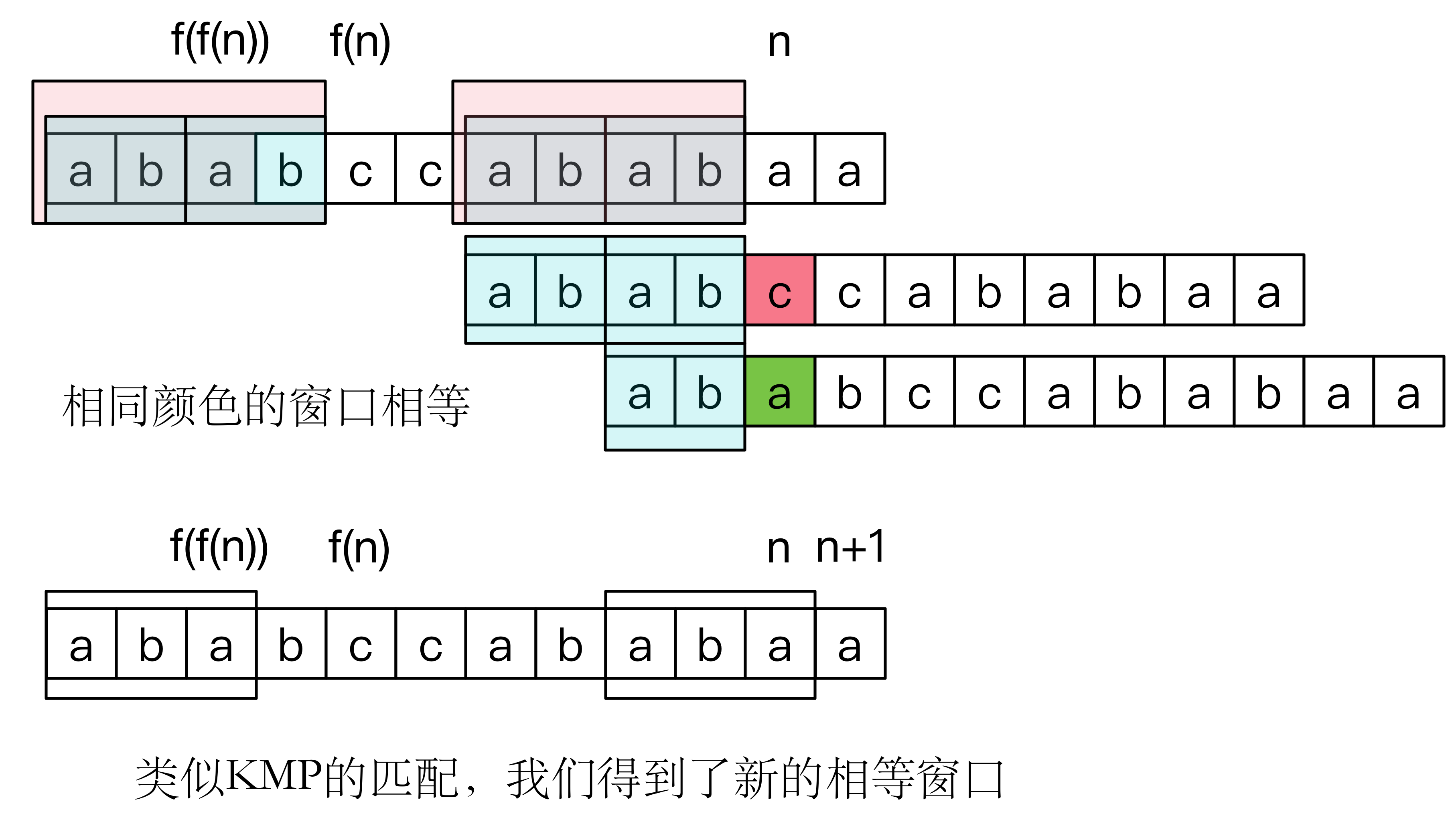void process(const char *temp, int *f) {
int n = strlen(temp);
f = f = 0; //边界
for(int i = 1; i < n; ++i) {
int j = f[i];
while(j && temp[i] != temp[j]) j = f[j]; //一旦回到1，表明窗口大小为0了，只能回到最初的字符
f[i + 1] = temp[i] == temp[j] ? j + 1: 0;
}
}



## 总结

KMP算法的精髓在于对已知信息的充分利用，这体现在待匹配串的匹配上面，更用于预处理时自己匹配自己上面，总而言之，KMP算法是非常值得学习的。